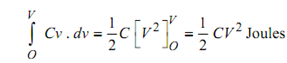## Energy Stored in a Capacitor Assignment Help

Assignment Help: >> Capacitance between two Parallel Plates - Energy Stored in a Capacitor

Energy Stored in a Capacitor:

Imagine the potential difference across a capacitor to be enhanced from V to (V + dv) volts in dt seconds.

We know                 i = C . (dv/ dt)

Instantaneous value of power to capacitor is

iv = vC . (dv / dt) watts

Energy supplied to capacitor during interval dt is

vC . (dv / dt ). dt = Cv . dv Joules

Hence, total energy when potential difference is increased from O to V volts is∴          W =( ½) CV 2